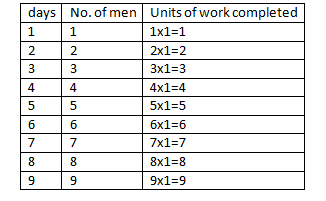# SSC CGL 2018 Practice Test Papers | Quantitative Aptitude (Day-36)

Dear Aspirants, Here we have given the Important SSC CGL Exam 2018 Practice Test Papers. Candidates those who are preparing for SSC CGL 2018 can practice these questions to get more confidence to Crack SSC CGL 2018 Examination.

[WpProQuiz 3279]

Click “Start Quiz” to attend these Questions and view Explanation

1) Which of the following numbers is divisible by both 24 and 15?

a) 758650

b) 459450

c) 268440

d) 584680

2) The present age of father is two years more than thrice his son’s age 10 years later. The age of the father ten years later will be 2 years more than 7 times his son’s present age. Find the present age of the father.

a) 60

b) 62

c) 70

d) 80

3) There are 2 numbers A and B. Five times the first, and four times the second are equal. Find the ratio of the squares of A and B.

a) 25/49

b) 4/9

c) 16/25

d) 25/36

4) A man employed a boy for 50 days on the condition that he will be paid Rs.2 for each day he works and will be fined rs.1 for each day he is absent.  At the end of 50 days, the boy received Rs.70 only. How many days was he absent?

a) 20

b) 10

c) 5

d) 25

5) The cost of an article is inversely proportional to the square root of the number of articles bought. If 81 articles are bought for 16 per article, what is the cost if 256 articles are bought?

a) 81

b) 16

c) 256

d) 9

6) Price of an article increases by 20% every year. If the difference between the price at the end of the third year and fourth year is rs.144, what was the price at the end of the second year?

a) 500

b) 600

c) 640

d) 560

7) In triangle ABC, AD is the angle bisector of CAB. If AC= 12 cm and AB= 8 cm, then what is the ratio of CD: BD?

a) 3:2

b) 2:3

c) 1:1

d) 1:3

8) Fifteen men can complete a job in three days. One of them started the job. At the end of each day one more man joined them. Find the number of days in which the job is completed?

a) 3

b) 6

c) 9

d) 11At the end of 9 days Total unit of work completed is= 1+2+3+4+5+6+7+8+9=45

9)  12 men can do a work in 15 days working hours a day. In how many days can 9 men do the same work, working 10 hours a day?

a) 12

b) 16

c) 20

d) 14

10) Find the roots of the equation x2-5x + 6 = 0

a) (2,3)

b) (-2,-3)

c) (1, 5)

d) (-1,5)

All the given 4 options are divisible by both 2 and 5.

Divisibility rule of 4= last 2 digits of the number should be divisible by 4

Divisibility rule of 3 = Sum of the digits in a number is divisible by 3

24 = 8×3 = 2 x 4 x 3

15 = 5 x 3

Let the present age of father and son be f and s respectively.

F=3(S+10) +2 = 3s + 32

F=3S+32 —— (i)

F+10=7S+2 ——- (ii)

Substituting f=3S+32 in (ii)

3S+32+10=7S+2

40 = 4S

S = 10 —- (iii)

Substitute (iii) in (i)

F = 3(10) + 32 = 62

A/B=4/5

A2/B2 = 16/25

Let the number of days present and absent be x and y respectively.

X + y = 50 —– (i)

2x – y = 70 —— (ii)

On solving (i) and (ii)

3x = 120

X = 40 —– (iii)

Substitute (iii) in (i) y = 10

C = k/√n

C1 = 16, n1 = 81

C2=? n2=256

C1√n1=k

C2√n2=k

16√81=C2√256

C2=9

Let the price at the end of the second year be Rs.100

The price at the end of the third year will be Rs.120 and fourth year will be 144

24 ——- 144

100 —— ?

?= 100×144/24=600

If AD is the bisector of CAB, then AC/AB = CD/DB

CD/DB =12/8 = 3/2

15 men can do a job in 3 days.

One man can do this job in 45 days.

Let the total work to be done is 45 units.

Efficiency of a man = 1 unit/dayAt the end of 9 days Total unit of work completed is= 1+2+3+4+5+6+7+8+9=45

M1D1H1=M2D2H2

12x15x8= 9xD2x10

D2=16 days

x2-5x+6=0

(x-2) (x-3)=0

X=2, 3